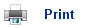# Atomic Sub Shells

• Atoms have electrons arranged in definite energy levels. The electrons within the first quantum shell are closest to the nucleus and have the lowest energies.
• Subsequent energy levels further away from the nucleus have higher energies.
• The shells are labelled by assigning each one a principal number, n, where n = 1, 2, 3ģ
• Evidence for electrons being arranged in energy levels is given by atomic spectroscopy and successive ionisation energy values.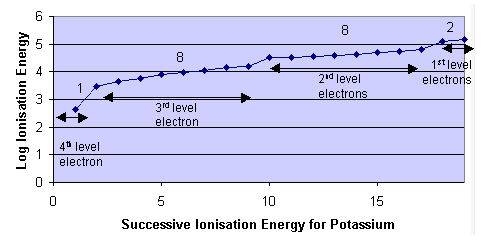• The energy levels are evident due to the large increase in ionisation energies after the removal of certain electrons.
• The number of electrons allowed in one energy level can be summarised by the formula 2n2 where n = energy level quantum number.
• Within energy levels in atoms, there are also sub energy levels, or orbitalss.
• The orbitals are labelled s, p, d and f. In the first energy level, there is only one orbital (s). The second shell has two orbitals (s and p), the third three (s, p, d) and the fourth has four (s, p, d, f).
• The different orbitals can hold a different number of electrons:
• 1 s orbital can hold 2
• 3 p orbitals can hold 6
• 5 d orbitals can hold 10
• 7 f orbitals can hold 14
• This explains why the total number of electrons in each energy level is 2n2.• The sub shells can be summarised in the diagram below, notice that the n=3 and n=4 sub shells overlap.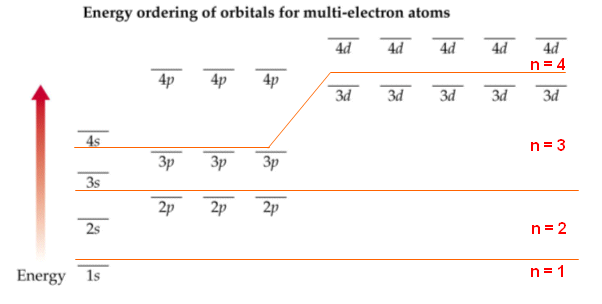## Atomic Orbitals

• The s, p, d and f s orbitals compose of regions of space where the electrons can be found.• Electrons within the same orbitals have the same amount of energy.
• The electrons do not lie within a specific place within an orbital, but can be found anywhere around the orbital.
• Each atomic orbital can contain 2 electrons, which can spin in two directions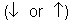, the electrons within the same orbital spin in opposite directions.
• To describe accurately the state of an electron, you need to be able to state:
1. The electron shell it is in (1, 2, 3, 4ģ.)
2. The orbital it is in within the electron shell (s, p, d, f)
3. Its spin.

## Filling the orbitals

• The arrangement of electrons within an atom, is referred to as the electronic configuration of an atom.
• The orbitals are filled within a definite order to produce the lowest energy arrangement available.
• The lowest energy levels are always filled first.
• Where there is more than one orbital within the same energy, the separate orbitals are filled separately with the same spin (think of bus analogy, i.e. people will aim to sit on their own seat on the bus, but when the seats are full, they will be forced to sit on a seat with someone else!). Then the electrons pair up in orbitals.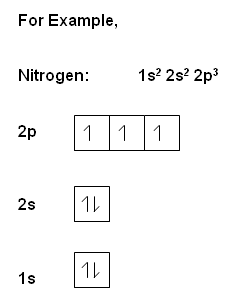## Useful books for revision:

Revise AS Chemistry for Salters (Written by experienced examiners and teachers of Salter's chemistry)Revise AS Chemistry for Salters (OCR) (Salters Advanced Chemistry)Home